Cisco CCNA How do you determine the mask to use?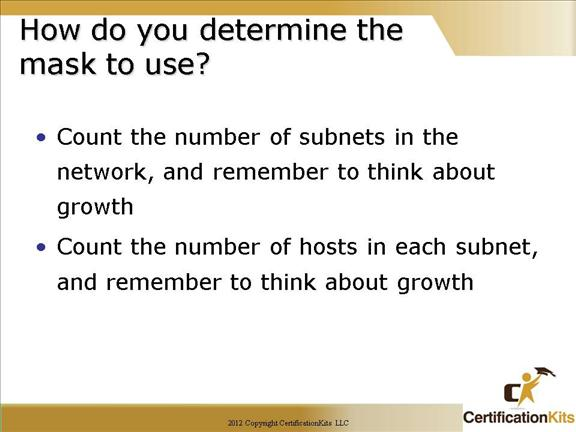1. Determine the number of required network IDs:

One for each subnet

One for each wide area network connection

2. Determine the number of required host IDs per subnet:

One for each TCP/IP host

One for each router interface

For example if you are provided a class C address and need to carve it up and are given requirements that you need one subnet to support 120 hosts, one subnet to support 50 hosts and four subnets to support 10 host each you can carve it up as follows:

Cisco CCNA After you choose a possible subnet mask…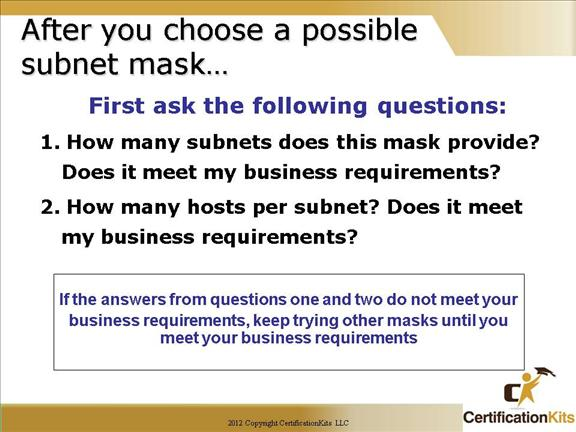This slide shows how to determine if a certain subnet mask will meet your business requirements of your Internetwork. It lists questions to ask when determining how to allocate IP addresses and subnets.  Remember to account for growth.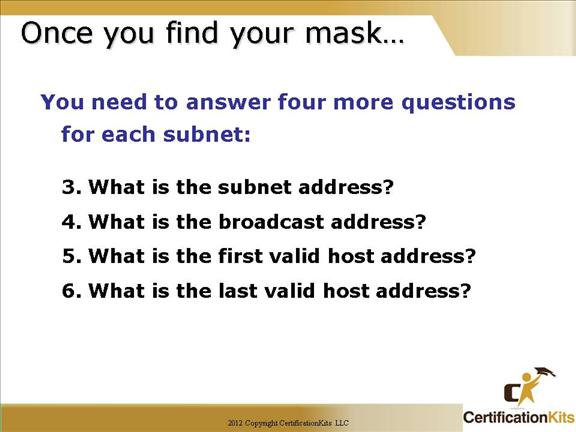Cisco CCNA Now, here is how to get Six Answers!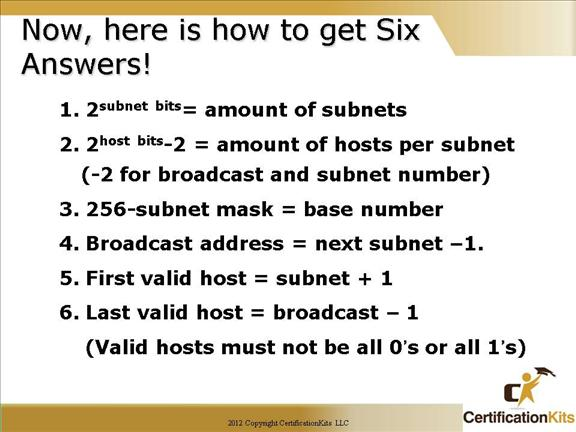This slide shows you how to achieve the answers to the six important subnetting questions:

How many subnets? 2x = number of subnets. x is the number of masked bits, or the 1s. For example, in 11000000, the number of ones gives us 22 subnets. In this example, there are 4 subnets.

How many hosts per subnet? 2x – 2 = number of hosts per subnet. x is the number of unmasked bits, or the 0s. For example, in 11000000, the number of zeros gives us 26 – 2 hosts. In this example, there are 62 hosts per subnet.

What are the valid subnets? 256 – subnet mask = block size, or base number. For example, 256 – 192 = 64. 64 is the first subnet. The next subnet would be the base number itself, or 64 + 64 = 128, (the second subnet).

You keep adding the base number to itself until you reach the value of the subnet mask, which is not a valid subnet because all subnet bits would be turned on (1s).

Whats the broadcast address for each subnet? The broadcast address is all host bits turned on, which is the number immediately preceding the next subnet.

What are the valid hosts? Valid hosts are the numbers between the subnets, minus all 0s and all 1s.

Cisco CCNA Classless Inter-Domain Routing
(CIDR)Another term you need to familiarize your self with is Classless Inter-Domain Routing (CIDR).

It is really just the method that ISP’s (Internet Service Providers) use to allocate an amount of addresses to a company, home—a customer.

They provide addresses in a certain block size—something we will be going into in greater detail later in this chapter.

So when you receive a block of addresses from an ISP, what you’ll get will look something like this: 192.168.10.32/28. What this is telling you is what your subnet mask is.

The slash notation (/) means how many bits are turned on (1’s). Obviously, the maximum could only be /32 because a byte is 8 bits and there are four bytes in an IP address: (4×8=32).   In the example, 192.168.10.32/28 means the address range provided including subnet and broadcast is 192.168.10.32 – 192.168.10.47.

But keep in mind that the largest subnet mask available (regardless of the class of address) can only be a /30 because you’ve got to keep at least two bits for host bits.

Cisco CCNA IP Subnet-Zero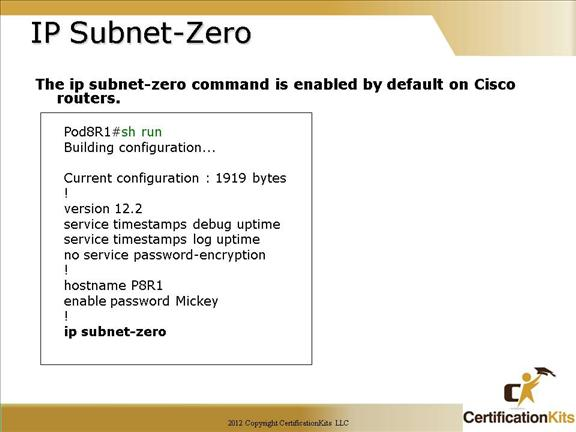The ip subnet-zero command provides the ability to configure and route to subnet 0 subnets.

Subnetting with a subnet address of 0 is discouraged because of the confusion inherent in having a network and a subnet with indistinguishable addresses. It has a major benefit in that it utilizes address space more efficiently.

Cisco CCNA What do we know?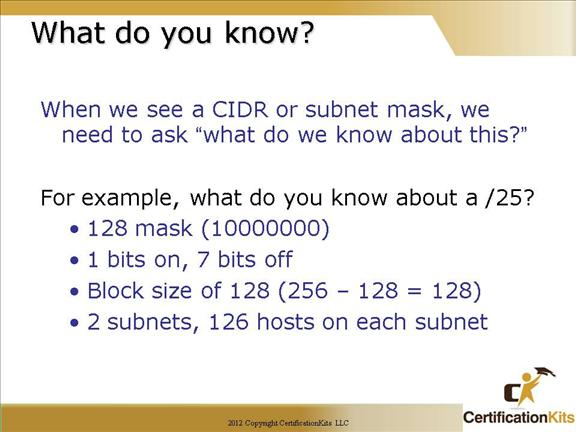This slide and the next few slides are added for your studying enjoyment and to help you memorize important class C subnetting information.

If you can remember this information, subnetting will be a breeze!

Cisco CCNA What do we know?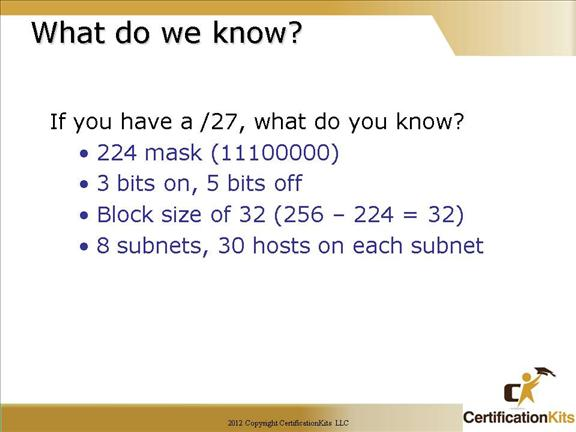Cisco CCNA What do we know?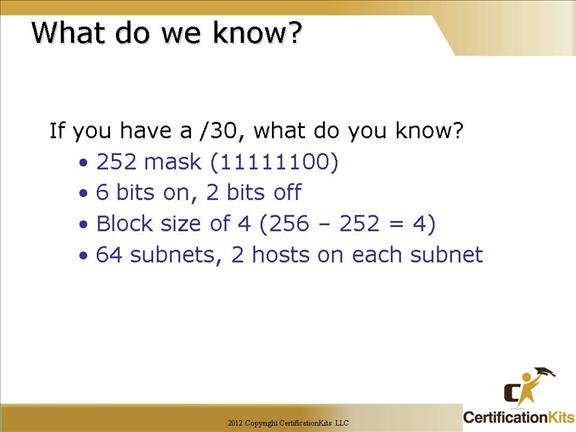Cisco CCNA Easy Subnetting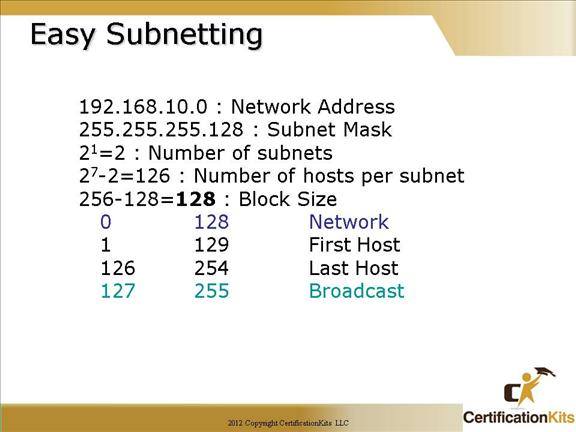How many subnets?

Since 128 is one bit on (10000000), the answer would be 21 = 2.

How many hosts per subnet? We have 7 host bits off (10000000), so the equation would be 27 – 2 = 126 hosts.

What are the valid subnets? 256 – 128 = 128, which our block size.  Start with 0 and keep adding the block size to get our valid subnets.

Our valid subnets are, then, 0 and 128.

Whats the broadcast address for each subnet? The number right before the next subnet is all host bits turned on and equals the broadcast address.

What are the valid hosts? These are the numbers between the subnets. The easiest way to find the hosts is to write out the subnet address and the broadcast address. This way the valid hosts are obvious.

Cisco CCNA Now, implement it…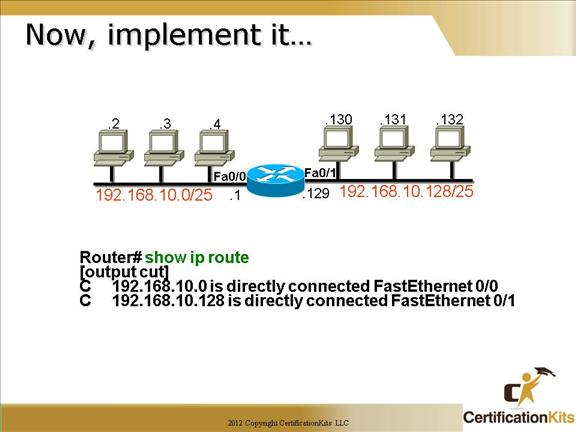This slide shows the output of a “show ip route” command when a Class C network is subnetted to a /25 (255.255.255.128) mask.

The routers can use any valid host address in the subnet, the hosts just need to know what it is so they can set the default gateway to that address.

The slide provides basic information on how you would implement a /25 Class C network and the routers routing table.  Each subnet supports 126 addresses per subnet, 128 total addresses (equals block size)  where the first address is for the network and the last address is the broadcast address.